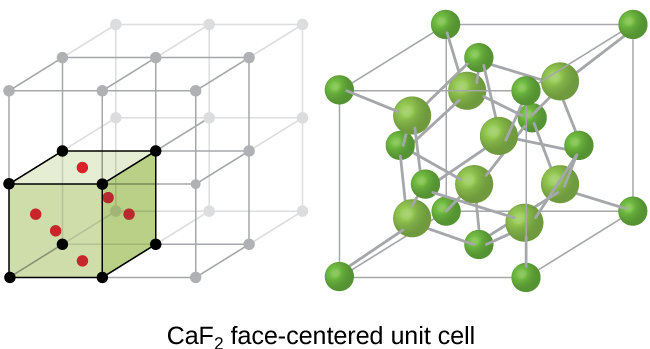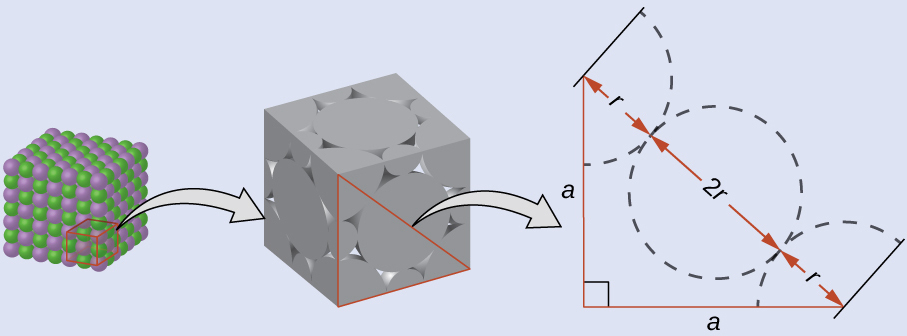# 10.6 Lattice structures in crystalline solids  (Page 8/29)

 Page 8 / 29Calcium fluoride, CaF 2 , forms an FCC unit cell with calcium ions (green) at the lattice points and fluoride ions (red) occupying all of the tetrahedral sites between them.

## Calculation of ionic radii

If we know the edge length of a unit cell of an ionic compound and the position of the ions in the cell, we can calculate ionic radii for the ions in the compound if we make assumptions about individual ionic shapes and contacts.

## Calculation of ionic radii

The edge length of the unit cell of LiCl (NaCl-like structure, FCC) is 0.514 nm or 5.14 Å. Assuming that the lithium ion is small enough so that the chloride ions are in contact, as in [link] , calculate the ionic radius for the chloride ion.

Note: The length unit angstrom, Å, is often used to represent atomic-scale dimensions and is equivalent to 10 −10 m.

## Solution

On the face of a LiCl unit cell, chloride ions contact each other across the diagonal of the face:Drawing a right triangle on the face of the unit cell, we see that the length of the diagonal is equal to four chloride radii (one radius from each corner chloride and one diameter—which equals two radii—from the chloride ion in the center of the face), so d = 4 r . From the Pythagorean theorem, we have:

${a}^{2}+{a}^{2}={d}^{2}$

which yields:

${\left(0.514\phantom{\rule{0.2em}{0ex}}\text{nm}\right)}^{2}+{\left(0.514\phantom{\rule{0.2em}{0ex}}\text{nm}\right)}^{2}={\left(4r\right)}^{2}=16{r}^{2}$

Solving this gives:

$r=\phantom{\rule{0.2em}{0ex}}\sqrt{\frac{{\left(0.514\phantom{\rule{0.2em}{0ex}}\text{nm}\right)}^{2}+{\left(0.514\phantom{\rule{0.2em}{0ex}}\text{nm}\right)}^{2}}{16}}\phantom{\rule{0.2em}{0ex}}=\text{0.182 nm}\phantom{\rule{0.2em}{0ex}}\left(\text{1.82 Å}\right)\phantom{\rule{0.2em}{0ex}}{\text{for a Cl}}^{\text{−}}\phantom{\rule{0.2em}{0ex}}\text{radius}.$

## Check your learning

The edge length of the unit cell of KCl (NaCl-like structure, FCC) is 6.28 Å. Assuming anion-cation contact along the cell edge, calculate the radius of the potassium ion. The radius of the chloride ion is 1.82 Å.

The radius of the potassium ion is 1.33 Å.

It is important to realize that values for ionic radii calculated from the edge lengths of unit cells depend on numerous assumptions, such as a perfect spherical shape for ions, which are approximations at best. Hence, such calculated values are themselves approximate and comparisons cannot be pushed too far. Nevertheless, this method has proved useful for calculating ionic radii from experimental measurements such as X-ray crystallographic determinations.

## X-ray crystallography

The size of the unit cell and the arrangement of atoms in a crystal may be determined from measurements of the diffraction of X-rays by the crystal, termed X-ray crystallography    . Diffraction is the change in the direction of travel experienced by an electromagnetic wave when it encounters a physical barrier whose dimensions are comparable to those of the wavelength of the light. X-rays are electromagnetic radiation with wavelengths about as long as the distance between neighboring atoms in crystals (on the order of a few Å).

When a beam of monochromatic X-rays strikes a crystal, its rays are scattered in all directions by the atoms within the crystal. When scattered waves traveling in the same direction encounter one another, they undergo interference , a process by which the waves combine to yield either an increase or a decrease in amplitude (intensity) depending upon the extent to which the combining waves’ maxima are separated (see [link] ).

#### Questions & Answers

to what volume must 8.32 NaOH be diluted to its analytical concentration 0.20 M
weight in mg 1.76 mole of I
Sheriza
the types of hydrocarbons
u are mad go and open textbook
Emmanuel
hahahahahahahahahahahahaha
Jessica
aliphatic and aromatic hydrocarbons
Osakue
I don't use to see the messages
how can you determine the electronegativity of a compound or in molecules
when u move from left to right in a periodic table the negativity increases
reeza
Are you trying to say that the elctronegativity increases down the group and decreases across the period?
Ohanaka
yes and also increases across the period
reeza
for instance when you look at one group of elements in a periodic table electronegativity decreases when you go across the table electronegativity increases. hydrogen is more electronegative than sodium, potassium of that group. oxygen is more electronegative than carbon.
reeza
i hope we all know that organic compounds have carbon as their back bone
OK,Thank you so much for the answer. I am happy now
can I ask you a question now
Osakue
yes
hanna
what is the oxidation number of nitrogen, oxygen and sulphur
Osakue
5, -2 & -2
hanna
What is an atom?
is a smallest particle of a chemical element that can exist
Osakue
can I ask a question
Osakue
it is a substance that cannot be broken down into simpler units by any chemical reaction
An atom is the smallest part of an element dat can take part in chemical reaction.
Idris
an atom is the smallest part of an element that can take part in a chemical reaction nd still retain it chemical properties
Precious
what are the branches of an atomic mass
Still waiting for answers for a very long time now
that question is very strong oooo
Osakue
most of the questions I asked wasn't answered what's the problem guys?
hi, there is no problems ooo
Osakue
between H2SO4 and HCL which is the strongest dehydrating agent ?
Ibirogba
HCl is the strongest dehydrating agent
Osakue
ᴡʜᴀᴛ ᴡɪʟʟ ᴏʙsᴇʀᴠᴇᴅ ɪғ ʟᴇᴀᴅ(ɪɪ)ɴɪᴛʀᴀᴛᴇs ɪs ᴀᴅᴅᴇᴅ ᴏɴ ᴛᴏ sᴏᴅɪᴜᴍ ɪᴏᴅɪᴅᴇ sᴏʟᴜᴛɪᴏɴ
what is the functional group of alkanals
can someone explain salt analysis properly
Frankyx
Find the number of calcium atoms present in a sample weighing 2.0*10 raise to the power of -3g
What does avogadro's law states?
what is chemistry?
Is a branch of science that deals with matter in relation to energy
Kata
permanent hardness of water can be removed by ?
***watertreatmentbasics.com/temporary-and-permanent-hardness-of-water/
caco3
Owoyomi
ʜᴏᴡ
Gawaar
What are the basic area in in chemistry
acid, base and salt
John
Why do people study chemistry
John
becuz chemistry is the study of elements, nature and etc
John
and also the study of matter and atom
John
is chemistry hard
the answer to that question depends on you
John
what kind of question are u asking fam?
Ibirogba
his asking if chemistry is hard
John
not really just a matter of you willing to study
OkorieByByBy DanielrosenbergerByByByByByBy